# mne.make_sphere_model#

mne.make_sphere_model(r0=(0.0, 0.0, 0.04), head_radius=0.09, info=None, relative_radii=(0.9, 0.92, 0.97, 1.0), sigmas=(0.33, 1.0, 0.004, 0.33), verbose=None)[source]#

Create a spherical model for forward solution calculation.

Parameters:
r0

Head center to use (in head coordinates). If ‘auto’, the head center will be calculated from the digitization points in info.

If float, compute spherical shells for EEG using the given radius. If ‘auto’, estimate an appropriate radius from the dig points in Info, If None, exclude shells (single layer sphere model).

info

The `mne.Info` object with information about the sensors and methods of measurement. Only needed if `r0` or `head_radius` are `'auto'`.

Relative radii for the spherical shells.

sigmasarray-like

Sigma values for the spherical shells.

verbose

Control verbosity of the logging output. If `None`, use the default verbosity level. See the logging documentation and `mne.verbose()` for details. Should only be passed as a keyword argument.

Returns:
sphereinstance of `ConductorModel`

The resulting spherical conductor model.

Notes

The default model has:

```relative_radii = (0.90, 0.92, 0.97, 1.0)
sigmas = (0.33, 1.0, 0.004, 0.33)
```

These correspond to compartments (with relative radii in `m` and conductivities σ in `S/m`) for the brain, CSF, skull, and scalp, respectively.

New in version 0.9.0.

## Examples using `mne.make_sphere_model`#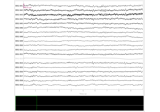Setting the EEG reference

Setting the EEG reference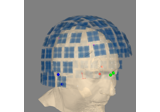Source alignment and coordinate frames

Source alignment and coordinate frames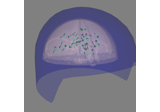Brainstorm Elekta phantom dataset tutorial

Brainstorm Elekta phantom dataset tutorial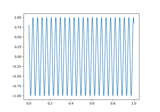Brainstorm CTF phantom dataset tutorial

Brainstorm CTF phantom dataset tutorial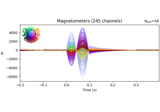4D Neuroimaging/BTi phantom dataset tutorial

4D Neuroimaging/BTi phantom dataset tutorial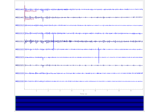Plot sensor denoising using oversampled temporal projection

Plot sensor denoising using oversampled temporal projection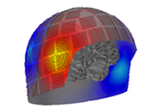Plotting sensor layouts of EEG systems

Plotting sensor layouts of EEG systems